# NCERT Solutions for Class 5 Maths How Big How Heavy## myCBSEguide App

CBSE, NCERT, JEE Main, NEET-UG, NDA, Exam Papers, Question Bank, NCERT Solutions, Exemplars, Revision Notes, Free Videos, MCQ Tests & more.

NCERT solutions for Class 5 Mathematics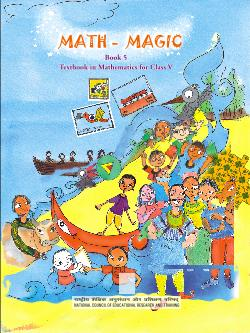## NCERT Solutions for Class 5 Maths How Big How Heavy

###### 1. A stage platform is made with 5 Math-Magic books. The volume of this stage is the same as ______ cm cubes.

Ans. 546 cm.

NCERT Solutions for Class 5 Maths How Big How Heavy

###### 2. Guess the volume of these things in cm cubes.

(a) A matchbox is about ___ cm cubes.

Ans. (a) 24 cm.

(b) A geometry box is about ___cm cubes.

Ans. (b) 90 cm.

(c) An eraser is about __ cm cubes.

Ans. (c) 6 cm.

###### 2. How will you check your guess?

Ans. In case of matchbox: It is about 6 cm long, 4 cm wide and 1 cm thick. So, 6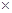41 i.e. 24 cm cubes will fit in it. Therefore, guess is correct.

In case of geometry box: It is about 16 cm long, 6 cm wide and 1 cm thick. So, 1661 i.e. 96 cm cubes will fit in it.

In case of eraser: It is about 3 cm long, 2cm wide and 1 cm thick. So, 321 i.e. 6 cm cubes will fit in it.

NCERT Solutions for Class 5 Maths How Big How Heavy

###### 3. Matchbox Play:

Tanu is making a stage with matchboxes. She first puts 14 matchboxes like this in the first layer. She makes 4 such layers and her stage looks like this.

(a) She used __ matchboxes to make this stage.

Ans. (a) 414 = 56.

(b) The volume of one matchbox is the same as 10 cm cubes. Then the volume of this stage is same as __ cm cubes.

Ans. (b) 560 cm.

(c) If all these cubes are arranged in a line, how long will that line be?

Ans. (c) 56 cm.

(d) Which has more volume- your Math-Magic book or Tanu’s platform?

Ans. (d) Tanu’s platform has more volume.

NCERT Solutions for Class 5 Maths How Big How Heavy

###### 4. With your friend’s collect many empty matchboxes of the same size. Measure the sides  and write here.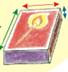Ans. My matchbox is 3 cm wide. It is 5 cm long. It is 1 cm high.

###### 5. Use 56 matchboxes to make platforms of different height. Fill this table.

Ans.

 How high is it? How long is it? How wide is it? Platform 1 4 layers 7 matchboxes 2 matchboxes Platform 2 2 layers 7 matchboxes 4 matchboxes Platform 3 1 layer 8 matchboxes 7 matchboxes

The volume of each platform if equal to 56 matchboxes.

###### 6. Mohan arranged his matchboxes like this.

How many matchboxes did he use to make it? What is its volume in matchboxes? ___ matchboxes.

Ans. Mohan first puts 44 = 2=16 matchboxes on the lower most layer. In the second he puts 33 = 9 matchboxes. In the third he puts 22 = 4 matchboxes and 1 matchboxes on the top most layer.

Total number of matchboxes used = (16 + 9 +4 +1) = 30

NCERT Solutions for Class 5 Maths How Big How Heavy

###### 7. How big is your cube?

(a) How long is the side of your cube?

Ans. (a) The side of the cube is 7 cm.

(b) How many centimetres cubes can be arranged along its:

Length? _______

Width? _______

Height? ______

Ans. (b) Number of centimetres cubes that can be arranged along its:

Length = 7 Width= 7 and Height =7.

To make the first layer on the table how many cm cubes will I use? How many such layers will I need to make?

Ans. (c) 49 cm cubes are needed for the first layer.

7 such layers are needed to make the cube.

(d) So the total cm cubes = ____

Ans. (d) The total cm cubes = 343.

(e) The volume of the paper cube is same as __ cm cubes.

Ans. (e) The volume of the paper cubes is same as 343 cm cubes.

###### 8. Anan made big cube having double the side of your paper cube. How many of the

your paper cubes will fit in it? Try doing by collecting all the cubes made in your class.

Ans. Side of Anan’s cube = 27 cm = 14 cm

In its first layer, we can arranged 22= 4 paper cubes (of side 7 cm). And 2 such layers of 4 paper cubes each can be packed. So, in Anan’s cube, we can arranged 42= 8 cubes.

NCERT Solutions for Class 5 Maths How Big How Heavy

###### 9.  Ganesh and Dinga went to pack 4000 centimetre cubes in boxes. These are to be sent to a school. There are three different boxes available for packing.

(a) What is your guess? Who is right?

Ans. (a) I think Ganesh is right.

(b) How can Ganesh and Dinga test their guesses before packing the cubes in the boxes? Discuss with your friend.

Ans. (b) Ganesh and Dinga should find the number of cubes to be fitted in the first layer and find the number of layers to fill the cubes. Their products gives us the total number of cubes that can be packed in each cube. In this way, they are able to check up their guesses.

(c) Use Ganesh’s method and write:

_____ centimeter cubes can be arranged in box B.

_____ centimeter cubes can be arranged in box C.

Ans. (c) In first layer of box B, we can arrange 1111 = 121 cubes. And 10 such layers of 121 cubes each can be packed. So, 12110 = 1210 centimetre cubes can be arranged in box B.

In first layer of box C, we can arrange 159 = 135 cubes. And 10 such layers of 135 cubes each can be packed. So, 13510 = 1350 centimetre cubes can be arranged in box C.

(d) So___ centimeter cubes in all can be packed in the three boxes.

Ans. (d) So, 2000 + 1210 + 1350 += 4560 centimetre cubes in all can be packed in the three boxes.

NCERT Solutions for Class 5 Maths How Big How Heavy

###### 10. (a) For 6 days, each person will need

Rice and flour __ g

Pulses ___ g

Dried onion __ g

Ans. (a) For 6 days, each person will need

Rice and flour = 6200 = 1200 g

Pulses =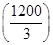= 400 g

Dried onions = 610 = 60 g

(b) How much of fresh tomatoes should be dried for 6 days for 10 people?

Ans. (b) Dried tomatoes need for 6 days for 10 people

= 61010 = 600 g

(c) What is the total weight of food (for 6 days) in each person’s bag?

Ans. (c) The total weight of food (for 6 days) in each person’s bag

= 1200 g + 400 g + 60 g + 506 g + 506 g + 406 g + 106g + 406g + 56g +    106g

= (1200 + 400 + 60 + 300 + 240 + 60 +240 + 30 + 60) = 2890 g

NCERT Solutions for Class 5 Maths How Big How Heavy

###### 11. Can you guess the weight of the heaviest animal on this Earth? No, it’s not me. I weigh only 5000 kg! It is the Blue Whale. Its weight is around 35 times more than me. So how many thousand kg does it weight?

Ans. The weight of the blue whale = 355000kg = 175000 kg = 175 thousand kg.

###### 12. Guess how many children of your weight will be equal to the weight of an elephant of 5000 kg.

Ans. Since my weight is 31 kg, I think that about 160 children of my weight will be equal to the weight of an elephant of 5000 kg.

NCERT Solutions for Class 5 Maths How Big How Heavy

###### 13. At birth, a baby elephant weighs around 90 kg. How much did you weigh when you were born? Find out. How many times is a baby elephant heavier than you were at birth?

Ans. Weight of a baby elephant at birth = 90 kg

My weight at the time of birth = 3 kg

Therefore, a baby elephant was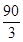= 30 times heavier than me at birth.

###### 14. If a grown up elephant eats 136 kg of food in a day than it will eat around __ kg in a month. Guess about how much it will eat in a year.

Ans. Weight of food eaten by a grown elephant in 1 day = 136 kg

Weight of food eaten by a grown elephant in 1 month i.e. 30 days = 13630 kg = 4080kg

Weight of food eaten by a grown elephant in a year i.e. 12 months

= 408012 kg = 48960 kg

NCERT Solutions for Class 5 Maths How Big How Heavy

###### 15.  Shahid works in a bank. He sits at the cash counter. Whenever there are too many coins he does not them. He just weighs them. Can you hold these coins and say which is the heaviest?

(1) How many coins are there in a sack of 5 rupees coins if it weigh:

(a) 18 kg?

Ans. (a) Since 1 kg = 1000 g. So, 18 kg = 18000 g.

If one coin weights 9g, then the sack weighing 18000 g has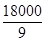= 2000 coins in it.

(b) 54 kg?

Ans. (b) Since 1 kg = 1000 g. So, 54 kg = 54000 g.

If one coin weights 9g, then the sack weighing 54000 g has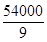= 6000 coins in it.

(c) 4500g?

Ans. (c) If one coin weighs 9g, then the sack weighing 4500 g has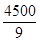= 500 coins in it.

(d) 2kg and 250g?

Ans. (d) 2 kg and 250 g = 21000g + 250 g = 2000 g + 250 g = 2250 g.

If one coin weighs 9g, then the sack weighing 2250 g has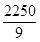= 250 coins in it.

(e) 1 kg and 125 g?

Ans. (e) 1 kg and 125 g = 11000 g + 125 g = 1000 g + 125 g = 1125 g

If one coin weighs 9g, then the sack weighing 4500 g has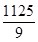= 125 coins in it.

(2) A 2 rupee coin weighs 6 g. What is the weight of a sack with:

(a) 2200 coins? ___ kg ____ g

Ans. (a) Weight of 2 rupee coins = 6 g

Weight of 2200 such coins = 22006 g = 13200 g = 13000 g + 200 g = 13 kg 200 g

(b) 3000 coins? ____ kg

If 100 one rupee coins weigh 485 g then how much will 10000 coins weigh? __ kg __ g.

Ans. (b) Weight of 2-rupee coins= 6 g

Weight of 3000 such coins =30006g = 18000 g = 181000 g = 18 kg

If 100 one-rupee coins weigh 485 g, then 10000 such coins weigh

= 485100 g = 48500 g = 481000 g = 500 g = 48 kg 500 g

## NCERT Solutions for Class 5 Mathematics Chapter 14 How Big How Heavy

NCERT Solutions Class 5 Maths PDF (Download) Free from myCBSEguide app and myCBSEguide website. Ncert solution class 5 Maths includes text book solutions from Class 5 Maths Book . NCERT Solutions for CBSE Class 5 Maths have total 14 chapters. 5 Maths NCERT Solutions in PDF for free Download on our website. Ncert Maths class 5 solutions PDF and Maths ncert class 5 PDF solutions with latest modifications and as per the latest CBSE syllabus are only available in myCBSEguide.

## CBSE app for Students

To download NCERT Solutions for Class 5 Maths, Social Science Computer Science, Home Science, Hindi English, Maths Science do check myCBSEguide app or website. myCBSEguide provides sample papers with solution, test papers for chapter-wise practice, NCERT solutions, NCERT Exemplar solutions, quick revision notes for ready reference, CBSE guess papers and CBSE important question papers. Sample Paper all are made available through the best app for CBSE students and myCBSEguide website.